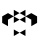Home
IT Knowledge
Inspiration
Languages
EN

# Python - boolean values

0 points
Created by:Hiba-Tate
248

In this article, we would like to show you boolean values in Python.

Boolean type has only two possible values:

1. `True`
2. `False`

## Practical examples

### Example 1

When you evaluate any expression in Python, you get the boolean value as a result - `True` or `False`.

In this example, we compare two values to receive a boolean result.

``````print(2 > 1)    # True
print(2 == 1)   # False
print(2 < 1)    # False``````

You can check the variable type using `type()` method:

``print(type(2 > 1))    # <class 'bool'>``

### Example 2

In this example, we use if statement to create a condition that returns the boolean value `True` or `False`. Based on the result, the program will print the appropriate sentence.

``````x = 1

if x > 0:
print("x is a positive number")  # if True
else:
print("x is a negative number")  # if False``````

Output:

``x is a positive number``